Next: Equilibrium of Constant-Temperature Constant-Pressure Up: Multi-Phase Systems Previous: Equilibrium of Isolated System

# Equilibrium of Constant-Temperature System

A knowledge of the equilibrium conditions for an isolated system permits us to deduce similar conditions for other situations of physical interest. For instance, much experimental work is performed under conditions of constant temperature. Thus, it would be interesting to investigate the equilibrium of some system,, in thermal contact with a heat reservoir,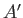, that is held at the constant absolute temperature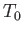.

The combined system,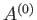, consisting of the systemand the heat reservoir, is an isolated system of the type discussed in the previous section. The entropy,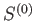, oftherefore satisfies condition (9.1). In other words, in any spontaneous process,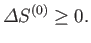(9.6)

However, this condition can also be expressed in terms of quantities that only refer to the system. In fact,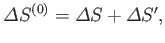(9.7)

where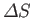is the entropy change of, and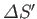that of the reservoir. But, ifabsorbs heat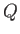from the reservoirduring the process thenabsorbs heat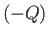, and suffers a corresponding entropy change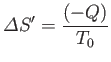(9.8)

(because it remains in equilibrium at the constant temperature). Furthermore, the first law of thermodynamics implies that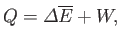(9.9)

where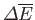is the change in internal energy of, and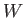is the work done by. Thus, Equation (9.7) can be written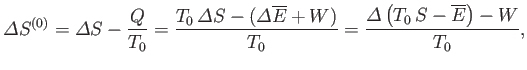(9.10)

or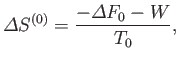(9.11)

where use has been made of the fact thatis a constant. Here,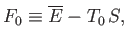(9.12)

reduces to the Helmholtz free energy,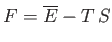, of system, when the latter has a temperature,, equal to that of the heat reservoir,. Of course, in the general case in whichis not in equilibrium with, the former system's temperature is not necessarily equal to.

The fundamental condition (9.6) can be combined with Equation (9.11) to give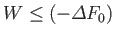(9.13)

(assuming thatis positive, as is normally the case). This relation implies that the maximum work that can be done by a system in contact with a heat reservoir is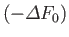. (Incidentally, this is the reason for the name free energy'' given to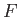.) The maximum work corresponds to the equality sign in the preceding equation, and is obtained when the process used is quasi-static (so thatis always in equilibrium with, and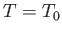). Equation (9.13) should be compared to the rather different relation (9.3) that pertains to an isolated system.

If the external parameters of systemare held constant then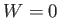, and Equation (9.13) yields the condition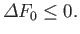(9.14)

This equation is analogous to Equation (9.1) for an isolated system. It implies that if a system is in thermal contact with a heat reservoir then its Helmholtz free energy tends to decrease. Thus, we arrive at the following statement:
If a system, whose external parameters are fixed, is in thermal contact with a heat reservoir then the stable equilibrium state is such that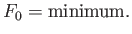The preceding statement can again be phrased in more explicit statistical terms. Suppose that the external parameters ofare fixed, so that. Furthermore, letbe described by some parameter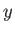. The thermodynamic functions of--namely,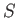and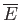--have the definite values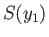and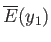, respectively, whenhas a given value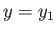. If the parameter changes to some other value,, then these functions change by the corresponding amounts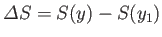and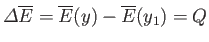. The entropy of the heat reservoir,, also changes because it absorbs heat. The corresponding change in the total entropy ofis given by Equation (9.11) (with):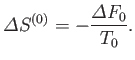(9.15)

But, in an equilibrium state, the probability,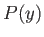, that the parameter lies betweenand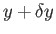is proportional to the number of states,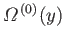, accessible to the isolated total system,, when the parameter lies in this range. Thus, by analogy with Equation (9.5), we have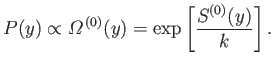(9.16)

However, from Equation (9.15),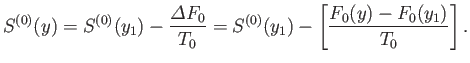(9.17)

Now, because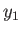is just some arbitrary constant, the corresponding constant terms can be absorbed into the constant of proportionality in Equation (9.16), which then becomes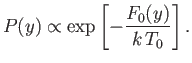(9.18)

This equation shows explicitly that the most probable state is one in which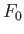attains a minimum value, and also allows us to determine the relative probability of fluctuations about this state.Next: Equilibrium of Constant-Temperature Constant-Pressure Up: Multi-Phase Systems Previous: Equilibrium of Isolated System
Richard Fitzpatrick 2016-01-25